Next: Dual Electromagnetic Field Tensor Up: Relativity and Electromagnetism Previous: Tensors and Pseudo-Tensors

# Electromagnetic Field Tensor

Let us now investigate whether we can write the components of the electric and magnetic fields as the components of some proper 4-tensor. There is an obvious problem here. How can we identify the components of the magnetic field, which is a pseudo-vector, with any of the components of a proper-4-tensor? The former components transform differently under parity inversion than the latter components. Consider a proper-3-tensor whose covariant components are written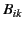, and which is antisymmetric: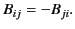(1762)

This immediately implies that all of the diagonal components of the tensor are zero. In fact, there are only three independent non-zero components of such a tensor. Could we, perhaps, use these components to represent the components of a pseudo-3-vector? Let us write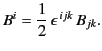(1763)

It is clear that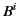transforms as a contravariant pseudo-3-vector. It is easily seen that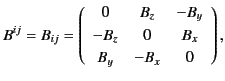(1764)

where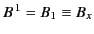, et cetera. In this manner, we can actually write the components of a pseudo-3-vector as the components of an antisymmetric proper-3-tensor. In particular, we can write the components of the magnetic field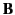in terms of an antisymmetric proper magnetic field 3-tensor which we shall denote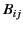.

Let us now examine Equations (1762) and (1763) more carefully. Recall that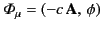and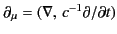. It follows that we can write Equation (1762) in the form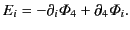(1765)

Likewise, Equation (1763) can be written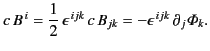(1766)

Let us multiply this expression by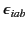, making use of the identity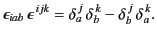(1767)

We obtain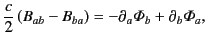(1768)

or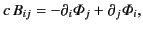(1769)

because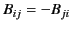.

Let us define a proper-4-tensor whose covariant components are given by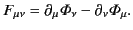(1770)

It is clear that this tensor is antisymmetric: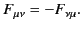(1771)

This implies that the tensor only possesses six independent non-zero components. Maybe it can be used to specify the components of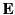and?

Equations (1767) and (1772) yield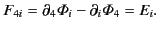(1772)

Likewise, Equations (1771) and (1772) imply that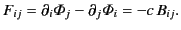(1773)

Thus,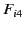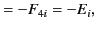(1774)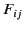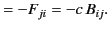(1775)

In other words, the completely space-like components of the tensor specify the components of the magnetic field, whereas the hybrid space and time-like components specify the components of the electric field. The covariant components of the tensor can be written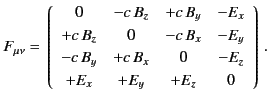(1776)

Not surprisingly,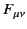is usually called the electromagnetic field tensor. The previous expression, which appears in all standard textbooks, is very misleading. Taken at face value, it is simply wrong. We cannot form a proper-4-tensor from the components of a proper-3-vector and a pseudo-3-vector. The expression only makes sense if we interpret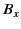(say) as representing the component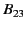of the proper magnetic field 3-tensorThe contravariant components of the electromagnetic field tensor are given by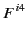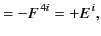(1777)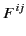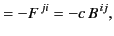(1778)

or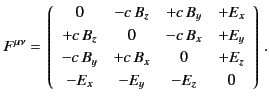(1779)

Let us now consider two of Maxwell's equations: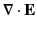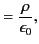(1780)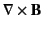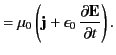(1781)

Recall that the 4-current is defined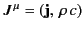. The first of these equations can be written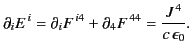(1782)

because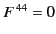. The second of these equations takes the form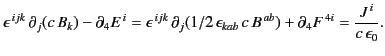(1783)

Making use of Equation (1769), the previous expression reduces to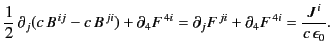(1784)

Equations (1784) and (1786) can be combined to give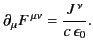(1785)

This equation is consistent with the equation of charge continuity,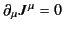, because of the antisymmetry of the electromagnetic field tensor.Next: Dual Electromagnetic Field Tensor Up: Relativity and Electromagnetism Previous: Tensors and Pseudo-Tensors
Richard Fitzpatrick 2014-06-27